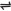## Ammonia and Its Properties

Ammonia molecules consist of one nitrogen and three hydrogen atoms, and has the formula, NH3.

Laboratory Preparation of Ammonia

Ammonia is prepared in the laboratory by the heating of a fine mixture of an ammonium salt (usually NH4Cl) and an alkali (usually Ca(OH)2).

Ca(OH)2(s) + 2NH4Cl(s) → CaCl2(s) + 2H2O(l) + 2NH3(g)

Note: the principle behind the reaction is this: more volatile alkalis, such as ammonia are liberated from their salts when less volatile alkalis act on them.

Hence, NH4Cl or NH4SO4 and Ca(OH)2, NaOH or KOH may be used together. The ammonia formed is dried using an unusual drying agent - calcium oxide, cao (quicklime).

This is because NH3 reacts with the usual drying agents: - It reacts with conc. H2SO4 to form a salt. Example,

2NH3(g) + H2SO4(l) → (NH4)2SO4(s)

It reacts with calcium chloride forming a complex solid compound, i.e.,

CaCl2(s) + 4NH3(g) → CaCl2 . 4NH3(s)

Commercial Production of Ammonia

Ammonia is made commercially by the direct combination of nitrogen and hydrogen using a catalyst - finely reduced (or divided) iron impregnated with aluminium oxide. A lower temperature of about 45oC and high pressure condition (between 200 to 500 atm are used.

This process is called the Haber process.

 N2(g) + 3H2(g)2NH3(g)

### Properties of Ammonia

Ammonia shows the following properties:

Physical Properties

1. Ammonia is a colourless gas.

2. It is less dense than air (v.d.= 8.5 - hence it is collected by upward delivery).

3. It is very soluble in water and is therefore used in the fountain experiment - it is a soluble gas

4. It is alkaline in nature, i.e., it is a soluble base, showing properties of alkalis.

Chemical Properties

1. Reaction with oxygen - it burns in oxygen with a greenish - yellow flame to form water vapour and nitrogen.

4NH3(g) + 3O2(g) → 6H2O(g) + 2N2(g)

In excess of oxygen, nitrogen oxide is formed instead of nitrogen.

4NH3(g) + 5O2(g) → 4NO(g) + 6H2O(g)

2. As a reducing agent - although NH3 is not a strong reducing agent, it reduces heated CuO, while it is itself oxidized to water and nitrogen.

3CuO(s) + 2NH3(g) → 3Cu(s) + 3H2O(l) + N2(g)

3. Reaction with chlorine - in excess ammonia, chlorine reacts with ammonia firstly to produce HCl and N2, then the excess NH3 react with the HCl to form white fumes of ammonium chloride.

2NH3(g) + 3Cl2(g) → 6HCl(g) + N2(g) then,

6NH3(g) + 6HCl(g) → 6NH4Cl(s) overall:

3Cl2(g) + 8NH3(g) → 6NH4Cl(s) + N2(g)

4. Reaction with carbon(IV)oxide - ammonia reacts with CO2 to produce urea, (NH2)2CO.

2NH3(g) + CO2(g) → (NH2)2CO(s) + H2O(l)

5. Thermal decomposition - at high temperature and prolonged sparking, ammonia decomposes to N2 and H2

6. As a precipitating agent - the hydroxides of most metals are precipitated from solutions of their salts by aqueous ammonia.

Example of such hydroxides are Fe(OH)3 - reddish brown; Pb(OH)2 - white; Cu(OH)2 - blue; and Zn(OH)2 - white gelatinous.

Note: in excess ammonia solution,Cu(OH)2 and Zn(OH)2 are dissolved. This can be used to identify both Cu and Zn ions. Cu(OH)2 dissolves to form a deep-blue complex compound, while Zn(OH)2 dissolves to form a colourless, complex compound.

### Test for Ammonia

Ammonia can be identified through the following tests:

• Smell - it has a characteristic choking smell.

• Action on litmus paper - turns moist, red litmus paper blue (It is alkaline).

• Reaction with conc. HCl - it forms a white fume NH3(g) + HCl(g) → NH4Cl(g)

### Uses of Ammonia

Here are ways by which ammonia can be used:

(1). Solution of ammonia is used in laundry work. It removes temporary hardness and removes also acids left on under clothing from evaporation of sweat.

(2). For the manufacture of trioxonitrate(V) acid.

(3). In the fertilizer industry - NH3 is used in the manufacture of urea which is used in the production of nitrogenous fertilizers, such as (NH4)2SO4 and ammonium tetra oxophosphate(V), (NH4)3PO4 - this is the most important use of ammonia.

(4). As refrigerant.

### Ammonium Salts and their Uses

Ammonium salts are electrovalent compounds, containing the ammonium ion, NH4+, in combination with a corresponding amount of an acidic ion, such as Cl-, NO3-, CO32- and SO42-. Ammonium salts of strong acids are hydrolyzed in their aqueous solutions to give acidic solutions.

This is because NH3 is a weak base. Action of heat on ammonium salts - ammonium salts are always decomposed by heat, and some of them sublime, that is, moving straight from solid to gas without going through the liquid state.

And when the gas is cooled, it solidifies without becoming liquid. A very good example is ammonium chloride, NH4Cl.

Note: ammonium salts from acids having a high proportion of oxygen are usually decomposed when heated. Example, ammonium dioxonitrate(III)

NH4NO2(s) → N2(g) + 2H2O(g) - Ammonium trioxonitrate(V)

NH4NO3(s) → N2O(g) + 2H2O(g)

Uses of Ammonium Salts

Ammonium salts has the following uses:

1. NH4Cl - two of the important uses of this salt are: (a). In the leclanche votaic cell. (b). As a flux in soldering.

2. (NH4)2SO4, NH4NO3 and (NH4)3PO4 are mainly used as fertilizers.

3. (NH4)2CO3 is used as a constituent of ‘smelting salts’, since it decomposes readily to ammonia, carbondioxide and water.

### Oxidation of Ammonia

Ammonia is oxidized to nitrogen(IV) oxide in the presence of excess air and platinum/rhodium gauze as catalyst.

The reaction is exothermic and the temperature of the system is maintained once it starts.

4NH3(g) + 5O2(g) → 4NO(g) + 6H2O(l)

The NO cools rapidly and combines with more oxygen (from the excess air) to form nitrogen(IV) oxide.

2NO(g) + O2(g) → 2NO2(g)

If the NO2 formed is passed into hot water, in the presence of more air, trioxonitrate(V) acid is formed.

2H2O(l) + 4NO2(g) + O2(g) → 4HNO3(aq)